# Decimals on number lines#### All in One Place

Everything you need for JC, LC, and college level maths and science classes.#### Learn with Ease

We’ve mastered the national curriculum so that you can revise with confidence.#### Instant Help

0/1
##### Intros
###### Lessons
1. Introduction to Decimals on Number Lines:
How to create decimal number lines for decimal tenths and decimal hundredths
0/13
##### Examples
###### Lessons
1. Reading decimals on number lines
What is the decimal given by the point on the number line?

1.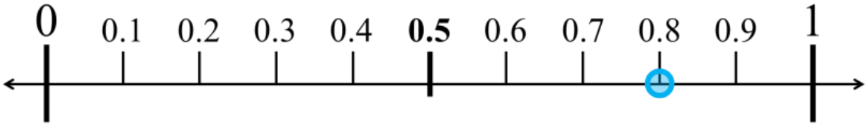2.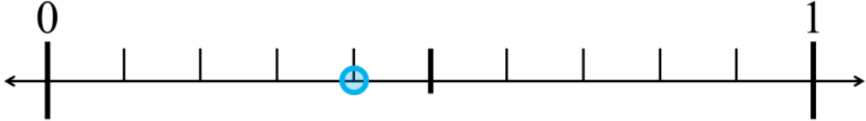3.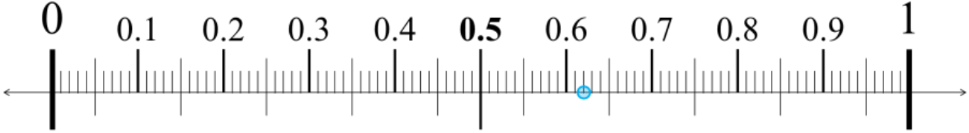4.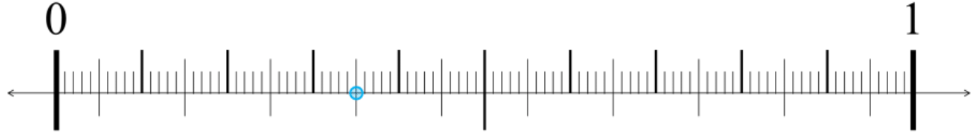2. Drawing decimals on number lines
Draw the decimal as a point on the number line

1.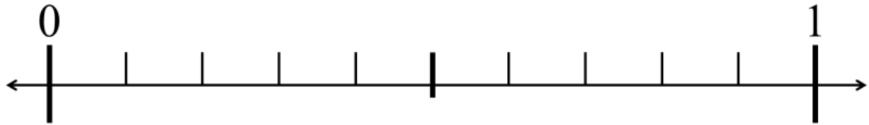2.3.4.3. Zooming into number lines and dividing into ten segments
Draw a point for the specified decimal. Label the number lines showing tenths and the zoomed in lines showing hundredths.

1.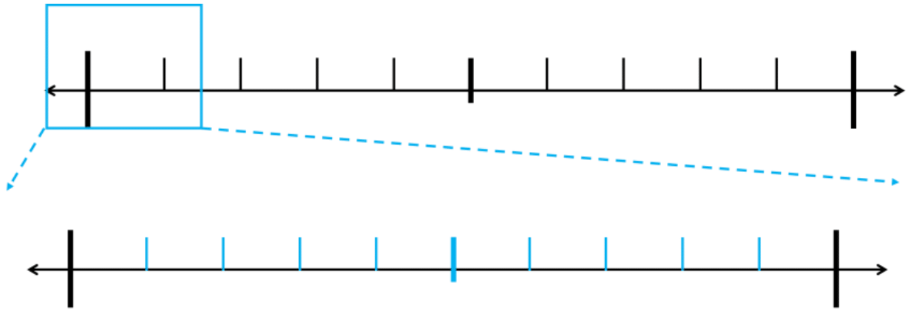2.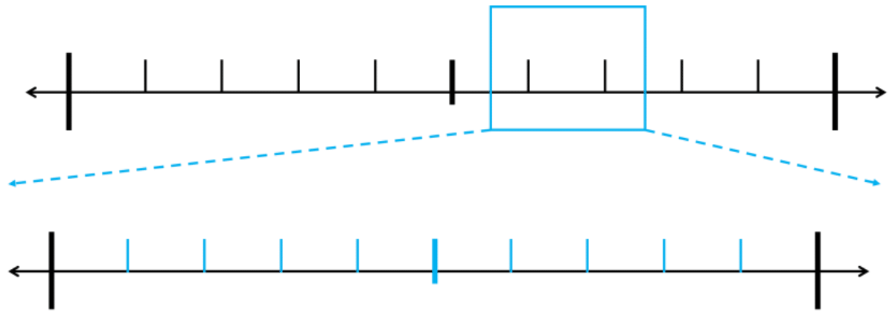3.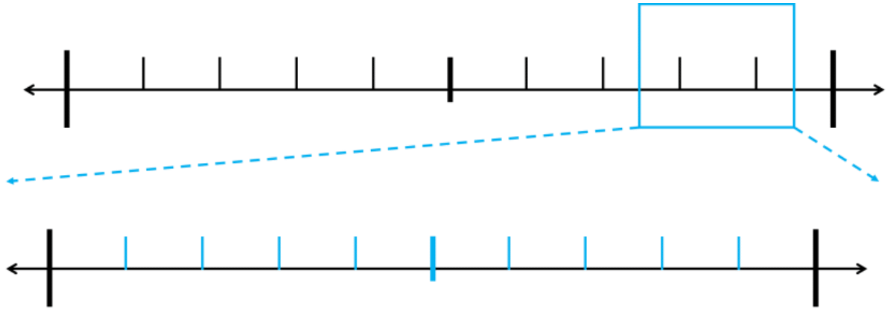4.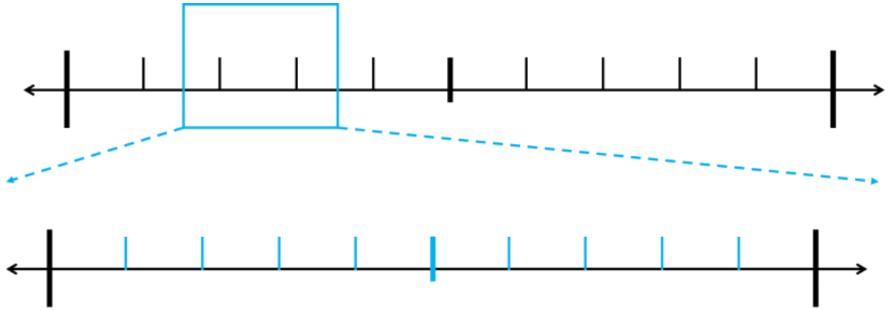5.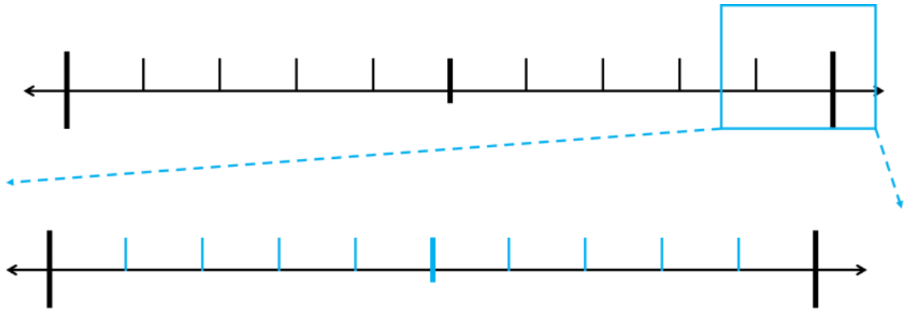0%
##### Practice
###### Topic Notes

In this lesson, we will learn:

• How to read number lines for decimals to the tenths or hundredths
• How to draw a point to represent a decimal on a decimal number line

Notes:

• A number line is a picture of a straight line with vertical markings of equal spacing
• Number lines increase from left to right (small $\, \longrightarrow \,$ big)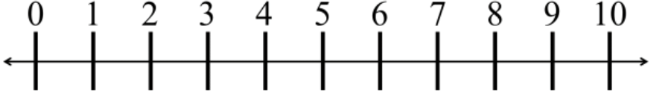• Decimals are numbers with place values even smaller than the ones place.
• Number lines can be divided even further between each number
• Splitting each division into 10 equal parts creates the next decimal place value
• Dividing the space between 0 to 1 into 10 equal parts, each part is a tenth
• Dividing the space between 0 to 0.1 into 10 equal parts, each part is a hundredth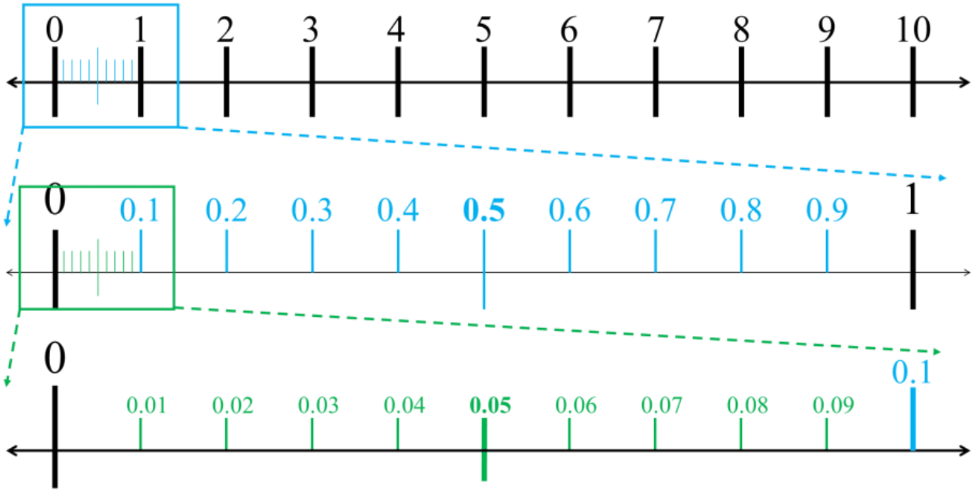• From 0 to 1, it is first split into 10 equal parts (tenths = 0.1)
• Each of these tenths 0.1 will be split into 10 more equal parts (10 tenths each into 10 parts = 10 × 10 = 100 parts)
• Ultimately, the space between 0 and 1 will be split into 100 equal parts
• That's why each 0.01 is called a hundredth (one of the 100 parts)